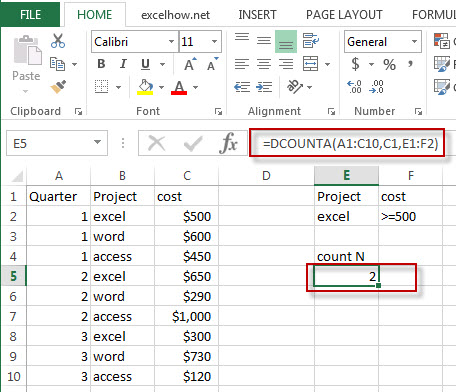Excel DcountA Function

This post will guide you how to use Excel DCOUNTA function with syntax and examples in Microsoft excel.

Description

The Excel DCOUNTA function returns the number of cells that containing nonblank values in a column of a list or a database that match the conditions that you specify.

The DCOUNTA function is a build-in function in Microsoft Excel and it is categorized as a Database Function.

The DCOUNTA function is available in Excel 2016, Excel 2013, Excel 2010, Excel 2007, Excel 2003, Excel XP, Excel 2000, Excel 2011 for Mac.

Syntax

The syntax of the DCOUNTA function is as below:

= DCOUNTA (database, field, criteria)

Where the DCOUNTA function arguments are:

• Database -This is a required argument.  The range of cells that containing the database.
• Field – This is a required argument.  The column to count the values.
• Criteria – The range of cells that contains the conditions that you specify.

Excel DCOUNTA Function Examples

The below examples will show you how to use Excel DCOUNTA Function to count the cells that contain nonblank values in a column of a list or database that match a given criteria.

#1 =DCOUNTA(A1:C10,C1,E1:F2)Note: The above excel formula will find excel project and counts how many of the cost fields in those records contains non-blank values.

Related Functions

• Excel Dcount Function
The Excel DCOUNT function returns the number of cells that containing numbers in a column of a list or a database that match the conditions that you specify.The syntax of the DCOUNT function is as below:= DCOUNT (database, field, criteria)…
Related Posts

Excel DVAR Function

This post will guide you how to use Excel DVAR function with syntax and examples in Microsoft excel. Description The Excel DVAR Function will get the variance of a population based on a sample of numbers in a column in ...

Excel DSUM Function

This post will guide you how to use Excel DSUM function with syntax and examples in Microsoft excel. Description The Excel DSUM Function will add the numbers in a column or database that meets a given criteria. And so you ...

Excel DSTDEVP Function

This post will guide you how to use Excel DSTDEVP function with syntax and examples in Microsoft excel. Description The Excel DSTDEVP returns the standard deviation of a population based on the entire population of numbers in a column or ...

Excel DSTDEV Function

This post will guide you how to use Excel DSTDEV function with syntax and examples in Microsoft excel. Description The Excel DSTDEV returns the standard deviation of a population based on a sample of numbers in a column or database ...

Excel DMIN Function

This post will guide you how to use Excel DMIN function with syntax and examples in Microsoft excel. Description The Excel DMIN returns the minimum value from a database or a column of a list that matches the specified conditions. ...

Excel DPRODUCT Function

This post will guide you how to use Excel DPRODUCT function with syntax and examples in Microsoft excel. Description The Excel DPRODUCT returns the product of values from a set of records that match criteria that you specify. The DPRODUCT ...

Excel Dmax Function

This post will guide you how to use Excel DMAX function with syntax and examples in Microsoft excel. Description The Excel DMAX returns the maximum value from a database or a column of a list that matches the specified conditions. ...

Excel Dget Function

This post will guide you how to use Excel DGET function with syntax and examples in Microsoft excel. Description The Excel DGET returns a single value from a database that matches the specified conditions. The DGET function is a build-in function ...

Excel Dcount Function

This post will guide you how to use Excel DCOUNT function with syntax and examples in Microsoft excel. Description The Excel DCOUNT function returns the number of cells that containing numbers in a column of a list or a database ...

Excel Daverage Function

This post will guide you how to use Excel DAVERAGE function with syntax and examples in Microsoft excel. Description The Excel DAVERAGE function calculates the average of values in a column of a list or database that match conditions you ...

Sidebar